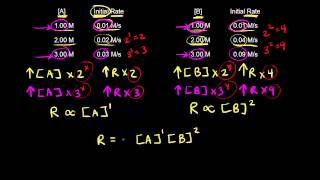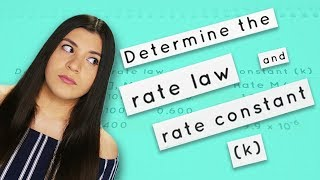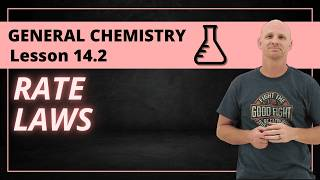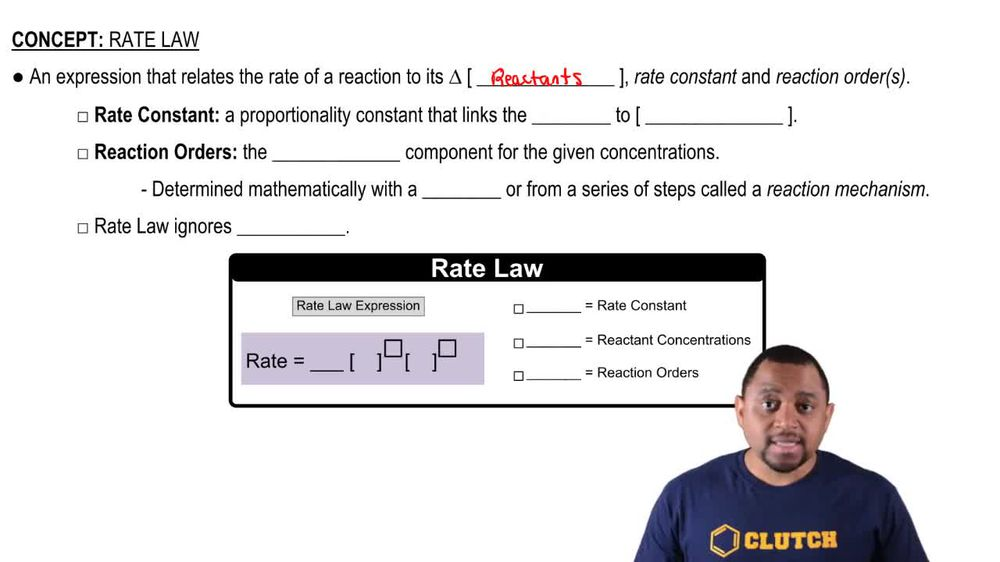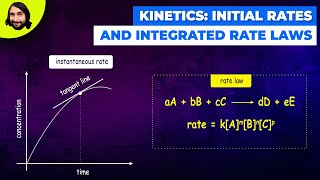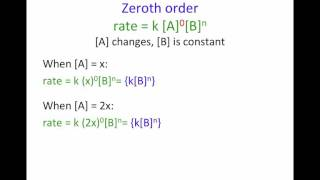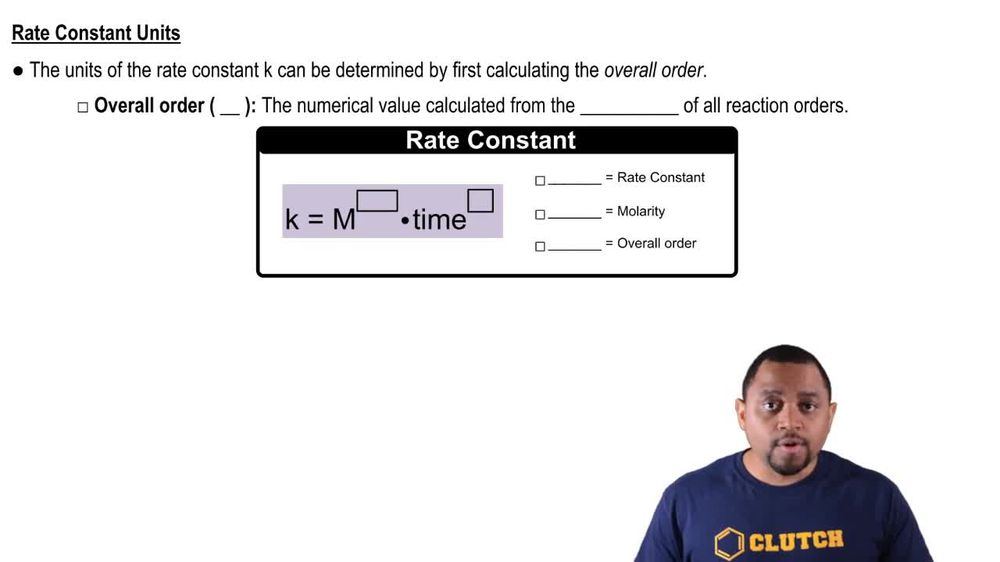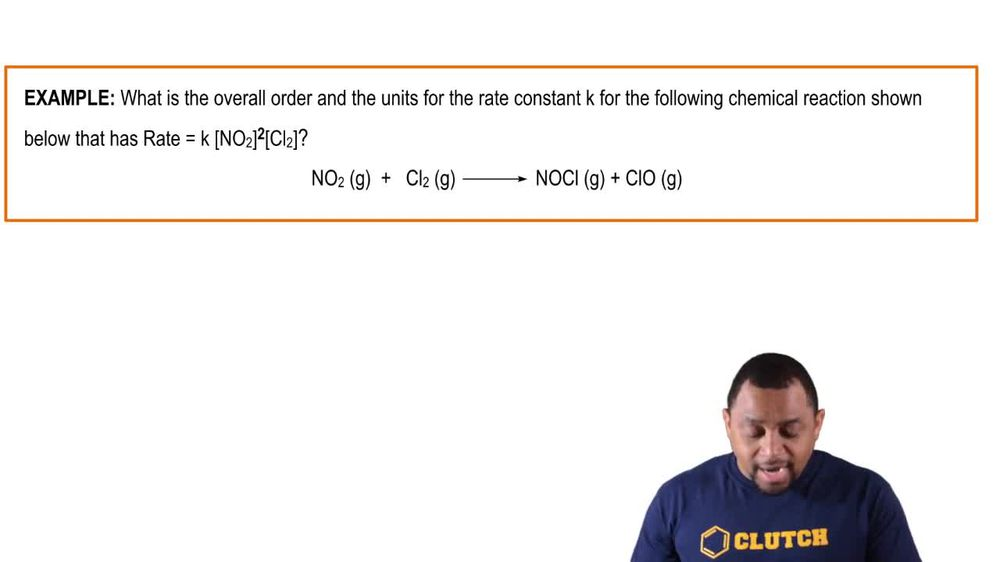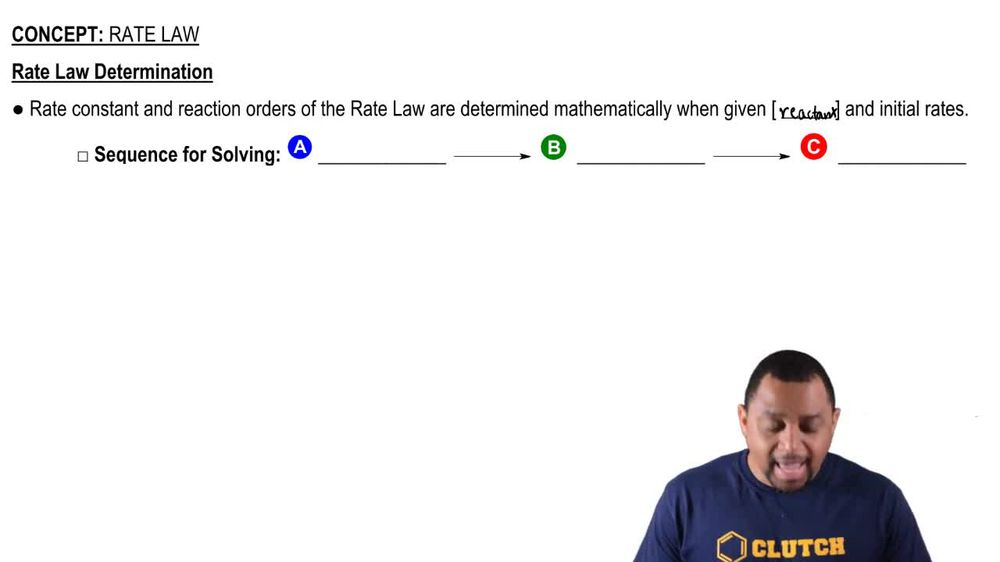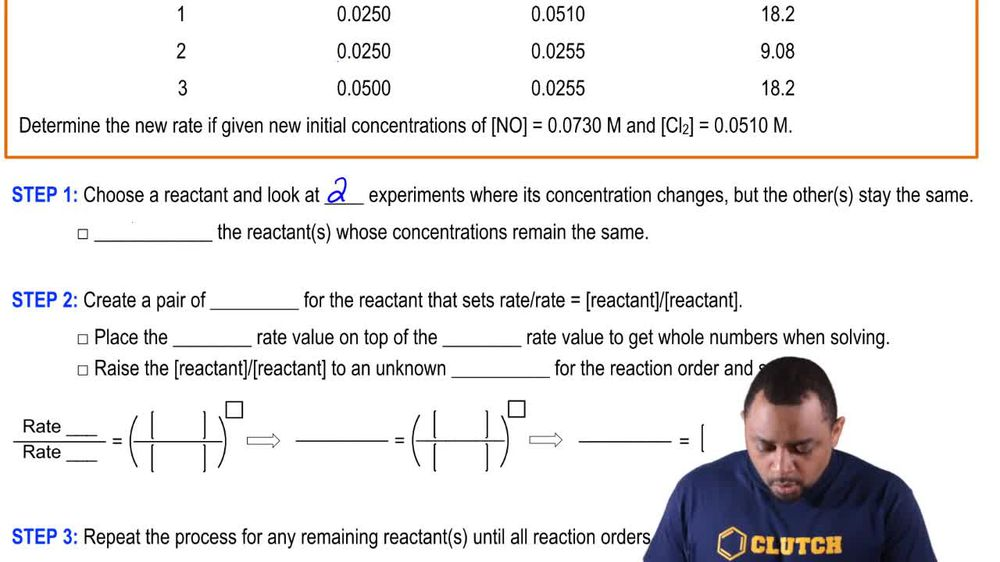Start typing, then use the up and down arrows to select an option from the list.
1. 15. Chemical Kinetics2. Rate Law# Rate Law Example 2

by Jules Bruno
164 views
0
what is the overall order? And the units for the rate constant K. For the following chemical reactions from below that has a rate equal to K N 02 to the two cl two to the one. Alright, so remember to and one are your reaction orders to find your overall order? You just add them together. So this would be two plus one. So your reaction would be third order overall. Now for K we'd say remember K is M to the negative end plus one times time inverse. Here, time could be in units of seconds, minutes, gears, whatever. So this would be adding to the negative three plus one. Here, let's just say seconds are the units of time that we're looking at? And so this would be m to the negative two times seconds inverse. So whatever the actual value of K would be, these would be the units that follow it. So it would be some value em to the negative two times s to the negative one. Okay, so we'd be third order overall. And these would be the units for our rate constant K.